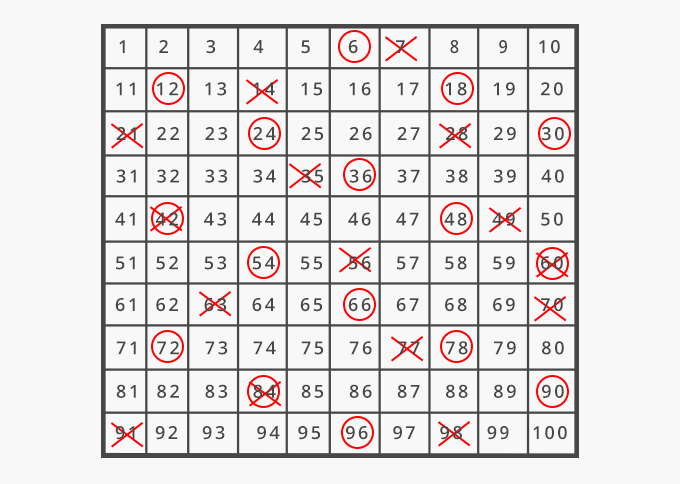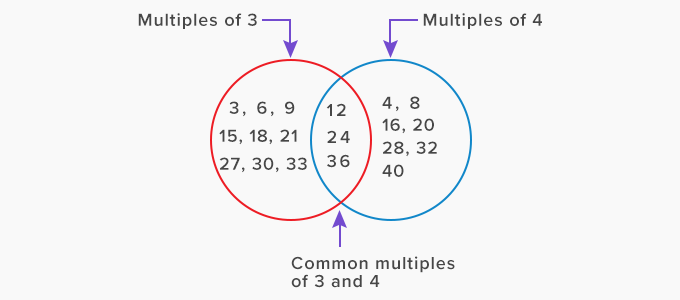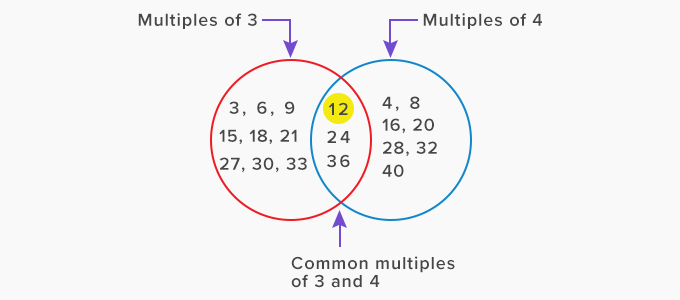# Common Multiple - Definition with Examples

The Complete K-5 Math Learning Program Built for Your Child

• 40 Million Kids

Loved by kids and parent worldwide

• 50,000 Schools

Trusted by teachers across schools

• Comprehensive Curriculum

Aligned to Common Core

What is Common Multiples?

Multiples

On multiplying a number by counting numbers,gives us its multiples.

For example, we can get the multiples of 6 and 7 by multiplying them by numbers 1, 2, 3, ... and so on.

Multiples of 6 are: 6, 12, 18, 24, 30, 36, 42, 48, 54, 60, …

Multiples of 7 are: 7, 14, 21, 28, 35, 42, 49, 56, 63, 70, ...

Common multiple

A common multiple is a whole number that is a shared multiple of each set of numbers. The multiples that are common to two or more numbers are called the common multiples of those numbers.

Let us mark the multiples of 6 and 7 on a hundreds grid. We will mark the multiples of 6 by a circle and multiples of 7 by a cross.The numbers that are circled as well as crossed are the common multiples of 6 and 7.

So, the common multiples of 6 and 7 are 42, and 84.

We can find the common multiples of two or more numbers by listing the multiples of each number and then finding their common multiples.

For example, to find the common multiples of 3 and 4, we list their multiples and then find their common multiples.

Multiples of 3: 3, 6, 9, 12, 15, 18, 21, 24, 27, 30, 33, 36, ...

Multiples of 4: 4, 8, 12, 16, 20, 24, 28, 32, 36, 40, ...

Common multiples of 3 and 4: 12, 24, 36, …Fun Facts A number can have an infinite number of multiples. Therefore, any two numbers or set of numbers can have the infinite number of common multiples.  The smallest common multiple of two or more numbers is called their Least Common Multiple (LCM). The LCM of 3 and 4 is 12.Won Numerous Awards & Honors